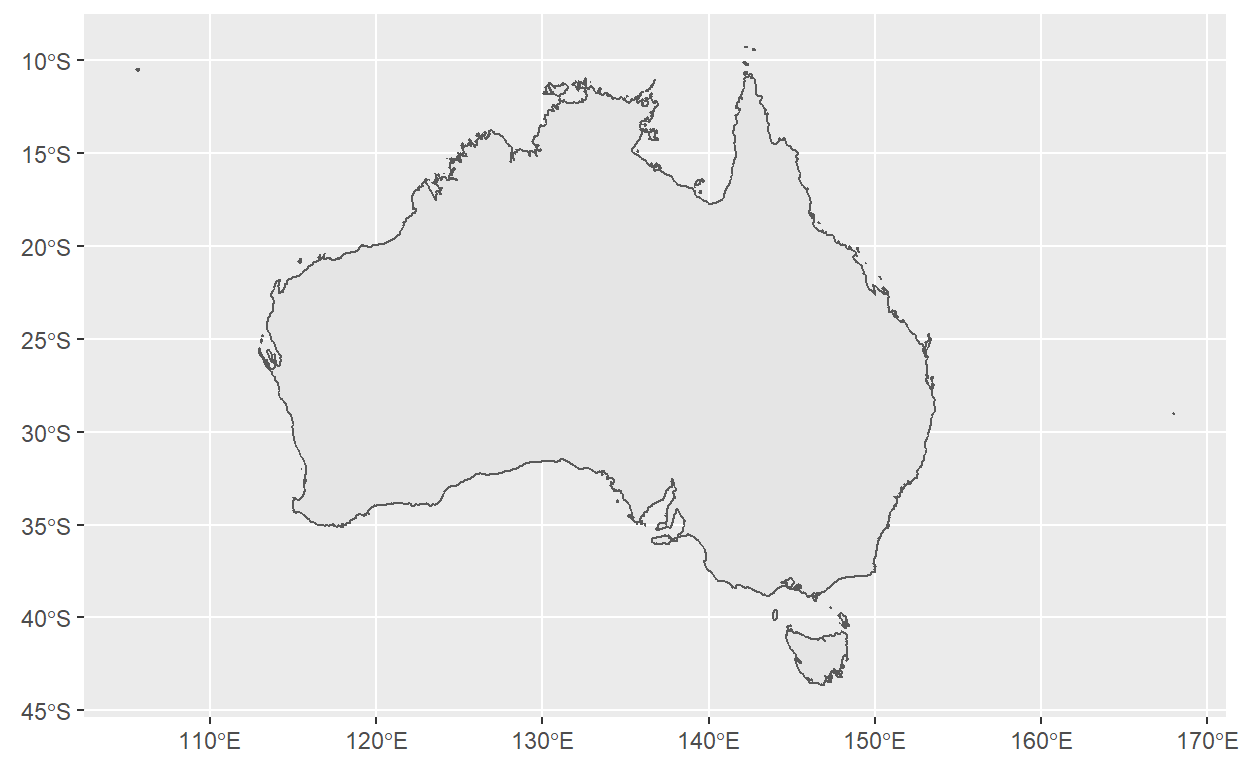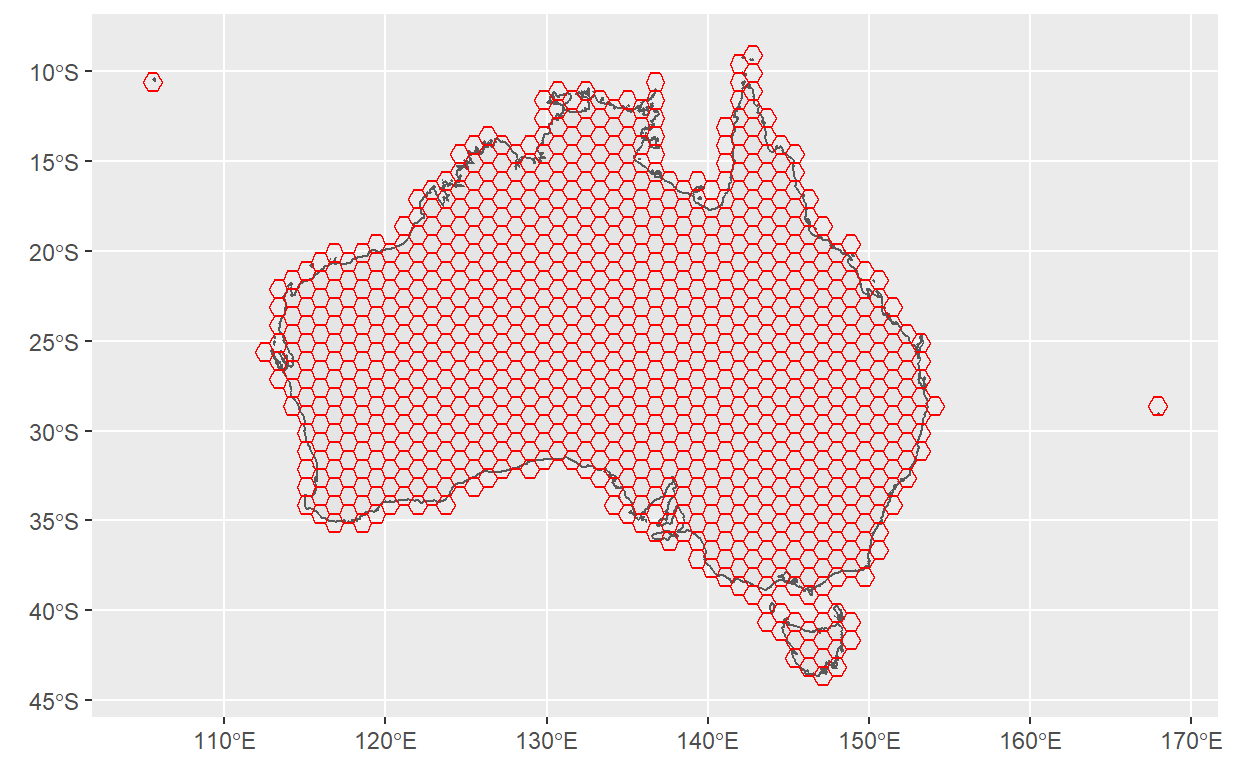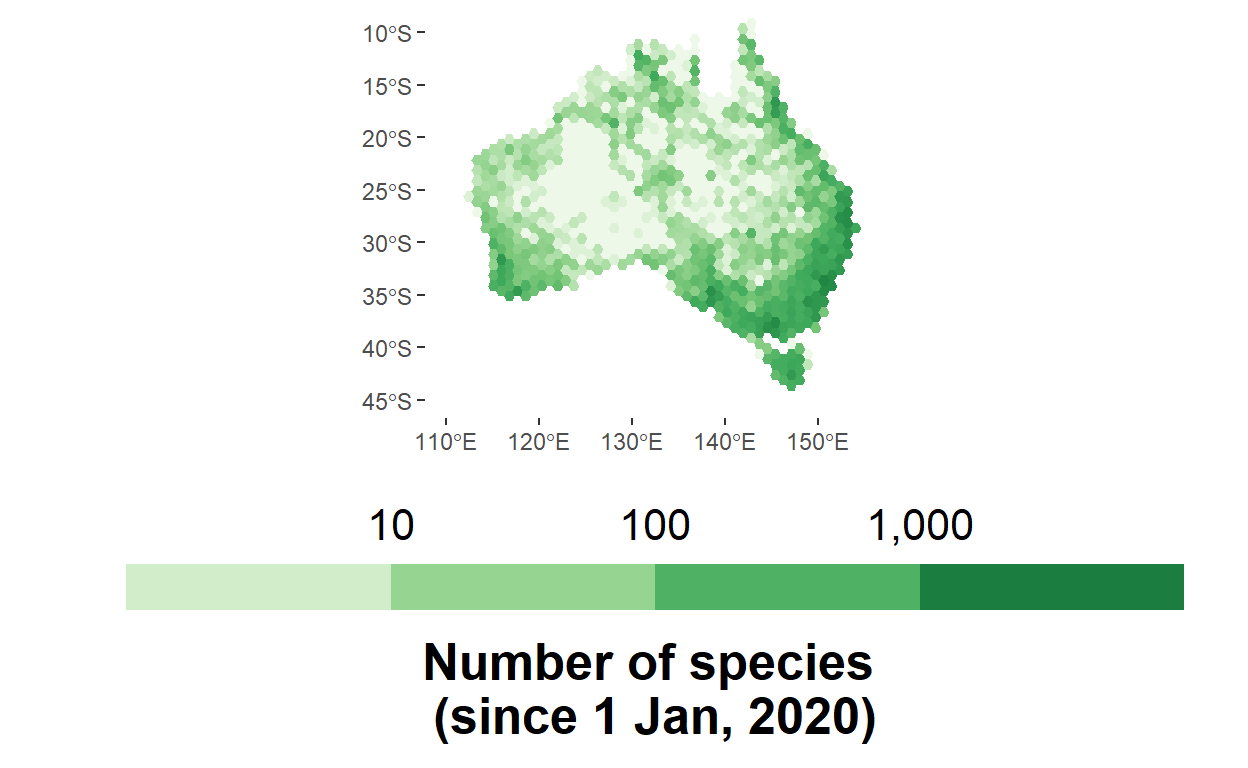# Download plant species data by hexagon to make a 3D hex map

Making plots eye-catching can be useful for science communication. Here, we show how to make 3D plots in R with the rayshader package by visualising the number of species identified from ALA observations since 2020

Dax Kellie
2022-05-23Dax Kellie

#### Date:

23 May, 2022

Grabbing people’s attention in a content-filled world can be difficult. 3D maps can be particularly eye-catching, and thanks to the rayshader package it has become relatively simple to make a beautiful 3D plot from a plot made with ggplot2.

In this post, we’ll make a 3D hex map of the number of plant species identified from ALA observations since 2020. This map builds on a previous hex map post, but this time we will use a more unique “grid-to-data” method to download our data, where instead of plotting hexagons over our map after extracting data, we’ll create a grid of hexagons that map to Australia before extracting any data and query the ALA for data for each hexagon. This method is cool because it saves a lot of work wrangling your data to fit your plot later on.

# Make a hexagon map

# packages
library(rayshader)  # For 3d rendering
library(tidyverse)  # Data wrangling
library(here)       # Safe paths
library(sf)         # Spatial features
library(ozmaps)     # For map of oz


Now let’s get a map of Australia from the ozmaps package

# get a map and project to WGS84
oz_wgs84 <- ozmap_data(data = "country") |>
st_transform(crs = st_crs("WGS84"))

## check map
ggplot(oz_wgs84) + geom_sf()Next let’s create our grid of hexagons and do some tidying to make sure the hexagons are only over the land

# create grid
oz_grid <- st_make_grid(oz_wgs84,
what = "polygons",
cellsize = 1.0,
square = FALSE,
flat_topped = TRUE)

# subset to grid cells that are within land
keep_hexes <- st_intersects(oz_grid, oz_wgs84)
keep_hexes <- as.data.frame(keep_hexes)$row.id oz_grid <- oz_grid[keep_hexes]  If we plot our new oz_grid over our map, we can see how the hexagons fill our map of Australia ## check ggplot() + geom_sf(data = oz_wgs84) + geom_sf(data = oz_grid, fill = NA, color = "red")# Download species data Now that we have our grid of hexagons, we can download data from the ALA using the galah package. Rather than downloading all data on the number of species identified since 2020 and then plotting the data as hexagons, we will make a function that sends individual queries to return the number of species identified within each hexagon. Our function get_counts() works in 3 parts: • The first part does some necessary editing of each Well Known Text (WKT) string so that they are compatible with galah. • The second part builds a query to download ALA data, beginning with galah_call(). We add the WKT for each hexagon to our query with galah_geolocate(), specify that we want to return only Plantae and Chlorophyta species with galah_identify(), and filter to only records from 2020 onwards with galah_filter(). We’ll also add galah_filter(profile = "ALA") to use a standard ALA data quality filter (known in the ALA as as a data “profile”). We end our query with atlas_counts(type = "species") to return counts of species, rather than counts of records (which is the default setting). • The final part makes sure that if any hexagons have 0 species identified, they will return a 0 rather than an NA, which triggers an error in R. get_counts <- function(hexagon){ # convert to wkt wkt_string <- st_as_text(oz_grid[[hexagon]]) %>% sub(")))", "))", .) %>% sub("POLYGON ", "POLYGON", .) # get counts result <- galah_call() |> galah_geolocate(wkt_string) |> galah_identify("plantae", "chlorophyta") |> galah_filter(profile = "ALA", decimalLongitude > 110, year >= 2020) |> atlas_counts(type = "species", # get species counts limit = NULL) # light formatting to catch errors if(is.null(result)){ tibble(count = NA, id = hexagon) }else{ result$id <- hexagon
result
}
}


We can use purrr::map() to run this function recursively for each hexagon. Then we can bind the separate lists into one data.frame with purrr::map_dfr(). As oz_grid is a spatial object containing POLYGONs (which R treats slightly differently to a data.frame), we have to use seq_along(oz_grid) to enable us to run the function for each line, which corresponds to each POLYGON.

IMPORTANT NOTE: This function will send lots of queries all at once to the ALA, so it is best to use restraint on how many times you run it because it can take a long time and, if run many times in a row, can make it take even longer.

# download number of species for each polygon
counts_list <- map(seq_along(oz_grid), get_counts)

# bind lists to data frame
counts_df <- map_dfr(counts_list, rbind)


counts_df now contains a single count of species for each hexagon, indicated by a unique id

head(counts_df, 10L)

# A tibble: 10 x 2
count    id
<dbl> <int>
1   396     1
2   303     2
3   457     3
4   178     4
5  1025     5
6   442     6
7   507     7
8   438     8
9   592     9
10     5    10

Now let’s merge our species counts in counts_df to our oz_grid hexagons so we can plot them. To do so, we’ll convert oz_grid to a tibble called oz_df, add a blank count column, and fill that column with the species counts in counts_df for each hexagon by id.

# convert to tibble, attach counts
oz_df <- st_as_sf(oz_grid)
oz_df$count <- NA oz_df$count[counts_df$id] <- counts_df$count


Let’s see the final result by checking the hexagons with highest species counts

# See top hexagons
oz_df %>%
arrange(desc(count)) %>%

Simple feature collection with 10 features and 1 field
Geometry type: POLYGON
Dimension:     XY
Bounding box:  xmin: 137.8823 ymin: -38.63203 xmax: 153.7594 ymax: -26.63203
Geodetic CRS:  WGS 84
count                              x
1   2543 POLYGON ((150.0066 -33.6320...
2   2144 POLYGON ((150.8726 -34.1320...
3   2073 POLYGON ((144.8105 -37.6320...
4   2005 POLYGON ((150.0066 -34.6320...
5   1959 POLYGON ((152.6047 -27.1320...
6   1949 POLYGON ((152.6047 -28.1320...
7   1804 POLYGON ((150.8726 -33.1320...
8   1616 POLYGON ((151.7387 -27.6320...
9   1604 POLYGON ((137.8823 -34.6320...
10  1577 POLYGON ((143.9444 -38.1320...

# Plot number of species

The first step to making our 3D map is to make a 2D map with ggplot2. I have set the fill of our map to use oz_df’s count column and log transformed it to make our final scale easier to read. The scale_fill_distiller() function has a nice “Greens” palette to make our plant species data look extra planty, and I have added custom limits and labels to make sure the scale is understandable.

hex_map <- ggplot() +
geom_sf(
data = oz_df,
mapping = aes(fill = log10(count + 1)), # log10 + 1 transformed
alpha = 1,
color = NA) +
scale_fill_distiller(name = "Number of species \n(since 1 Jan, 2020)",
type = "seq",
direction = 1,
limits = c(0,4),
labels = c("10", "100", "1,000"),
palette = "Greens",
# edit legend to be horizontal-bottom
guide = guide_colorsteps(direction = "horizontal",
label.position = "top",
title.position = "bottom",
title.hjust = 0.5)
) +
geom_sf(data = oz_wgs84,
color = NA,
fill = NA)  +
# crop map
coord_sf(xlim = c(110, 155),
ylim = c(-45, -10)) +
# Adjust text and make aesthetic more minimal
theme(title = element_text(face = "bold"),
legend.title = element_text(size = 19),
legend.position = "bottom",
legend.key.width = unit(28, 'mm'),
legend.text = element_text(size = 16),
plot.background = element_rect(fill = 'white', colour = 'white'),
panel.background = element_rect(fill = 'white', colour = 'white'),
axis.title = element_blank()
)

hex_map# Render in 3D

It’s time to get 3-Dimensional! Using rayshader::plot_gg(), we can render a nice 3d version of our plot1

# Render 3d plot
plot_gg(hex_map,
width = 9,
height = 8,
scale = 300, # adjust height of 3D transformation
windowsize = c(1200, 960), # adjust window of rendered plot
fov = 75,    # adjust size/strength of blur around outer edges
zoom = 0.37,
theta = 320, # adjust left-right rotation of view
phi = 33)    # adjust height of view